# RD Sharma Solutions for Class 6 Maths Chapter 16: Understanding Three Dimensional Shapes Exercise 16.1

We come across various Three-Dimensional Shapes in our daily lives and having a basic idea about them is very important. Exercise 16.1 has problems based on shapes like cuboid and cube. The objects like matchbox, tea packet, chalk box, dice and book etc. are the examples of cuboid and wooden box is an example of a cube. Subject matter experts design solutions based on the understanding capacity of students irrespective of their intelligence quotient. Students can refer RD Sharma Solutions Class 6 Maths Chapter 16 Understanding Three Dimensional Shapes Exercise 16.1 PDF which are provided here.

## RD Sharma Solutions for Class 6 Maths Chapter 16: Understanding Three Dimensional Shapes Exercise 16.1 Download PDF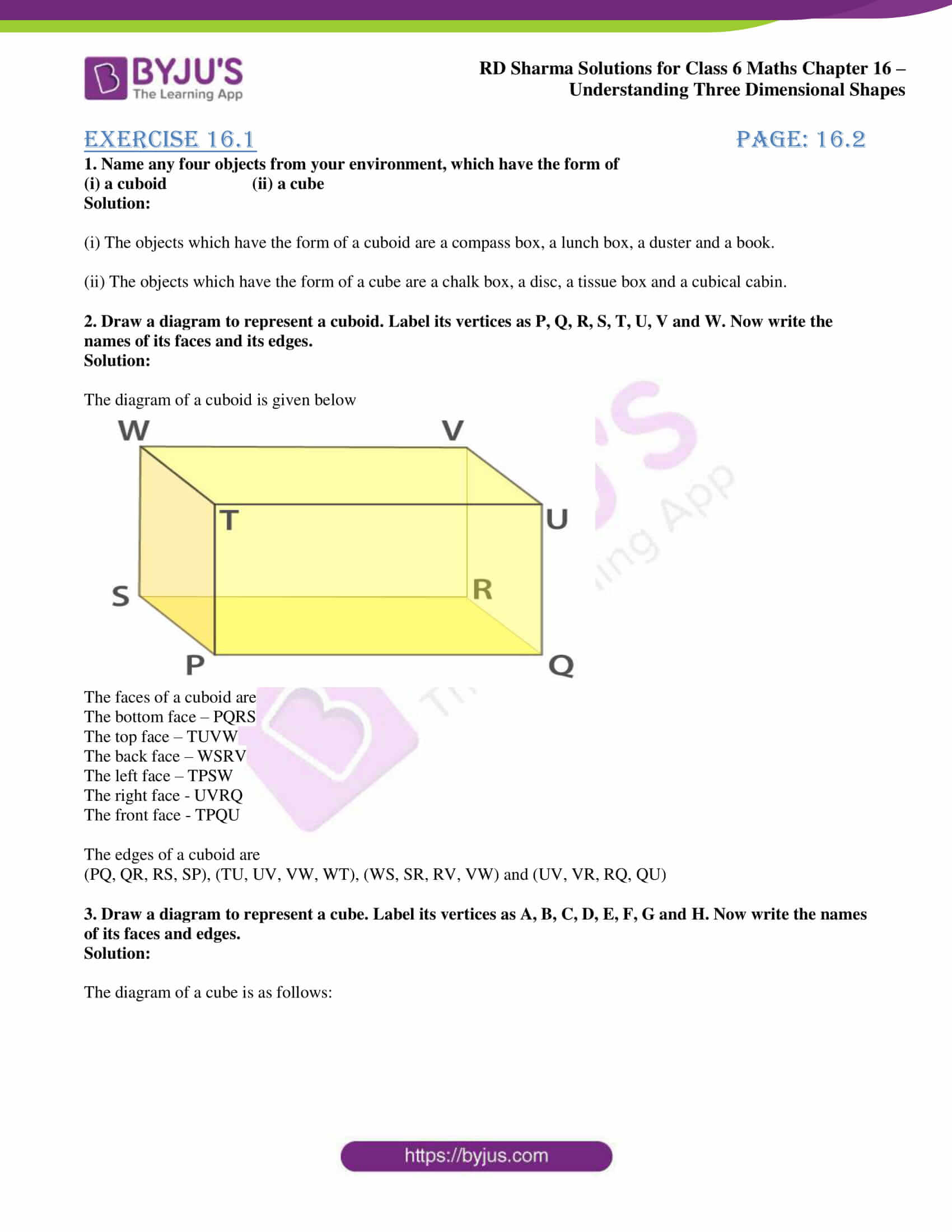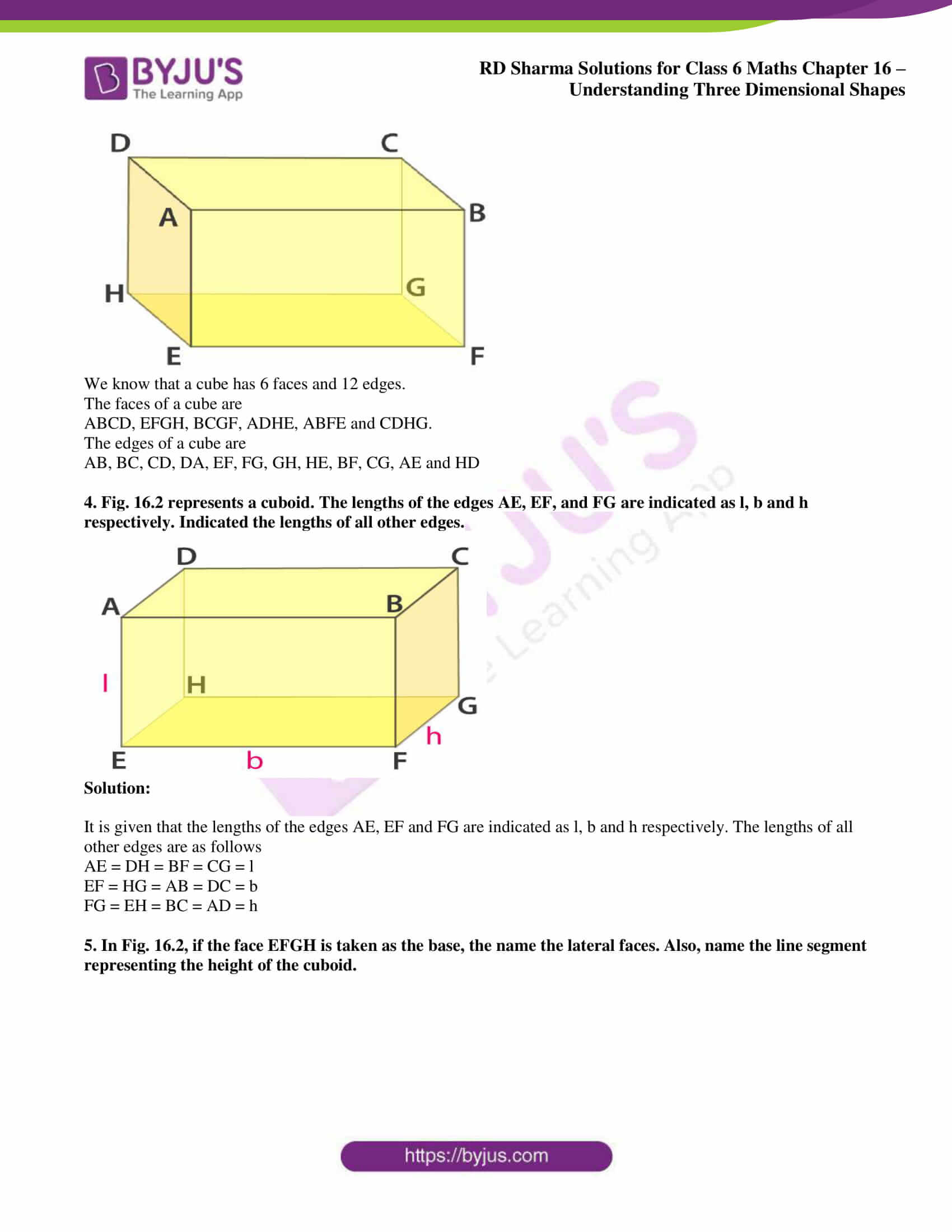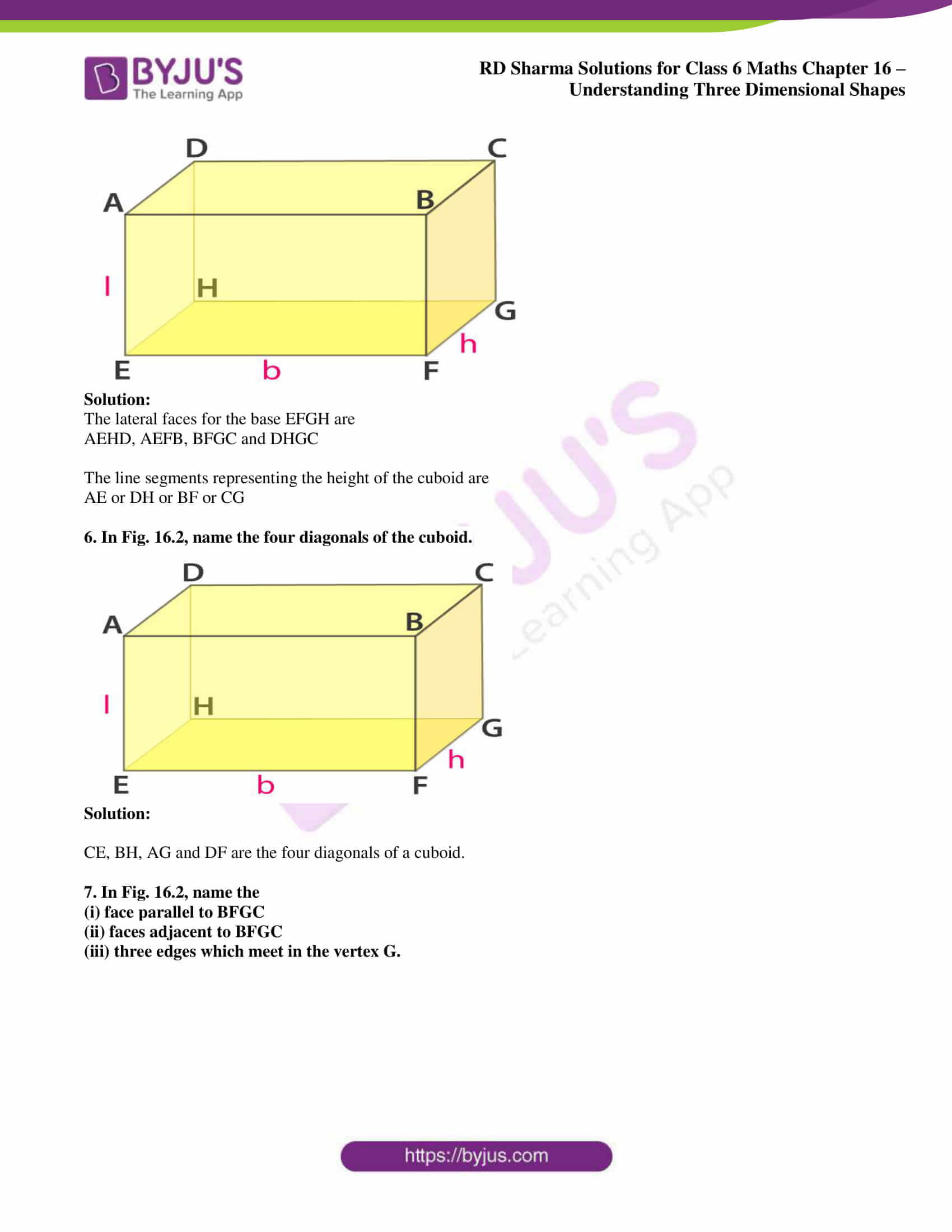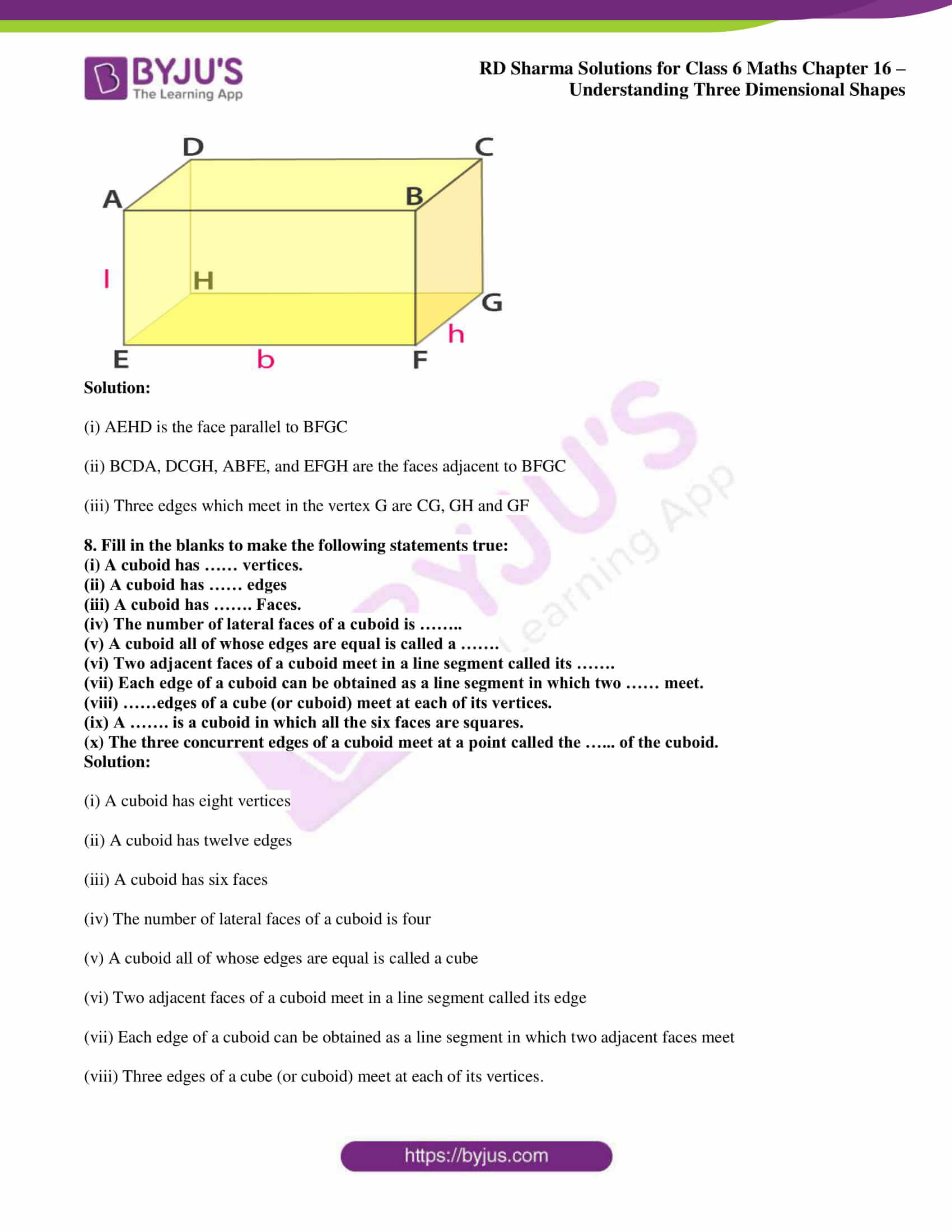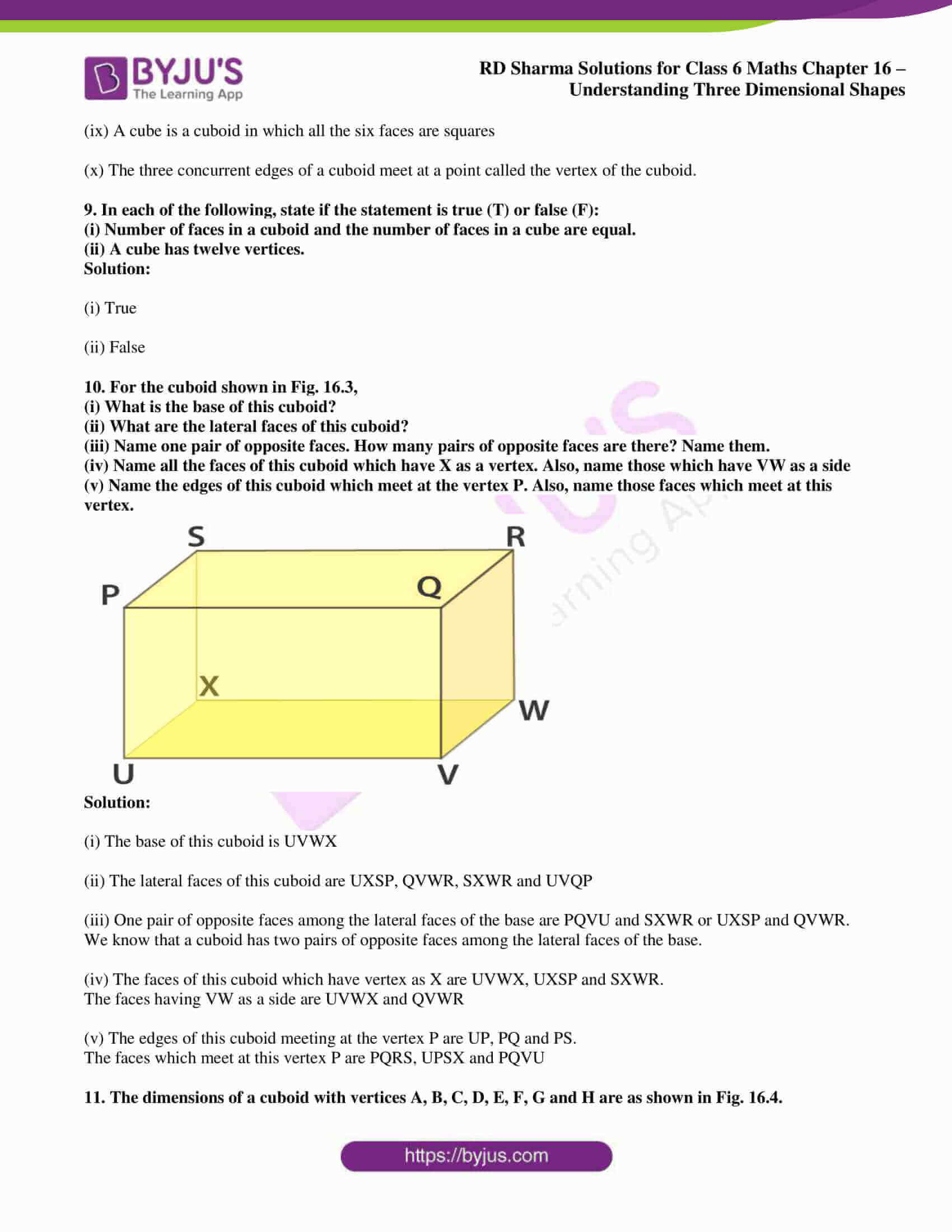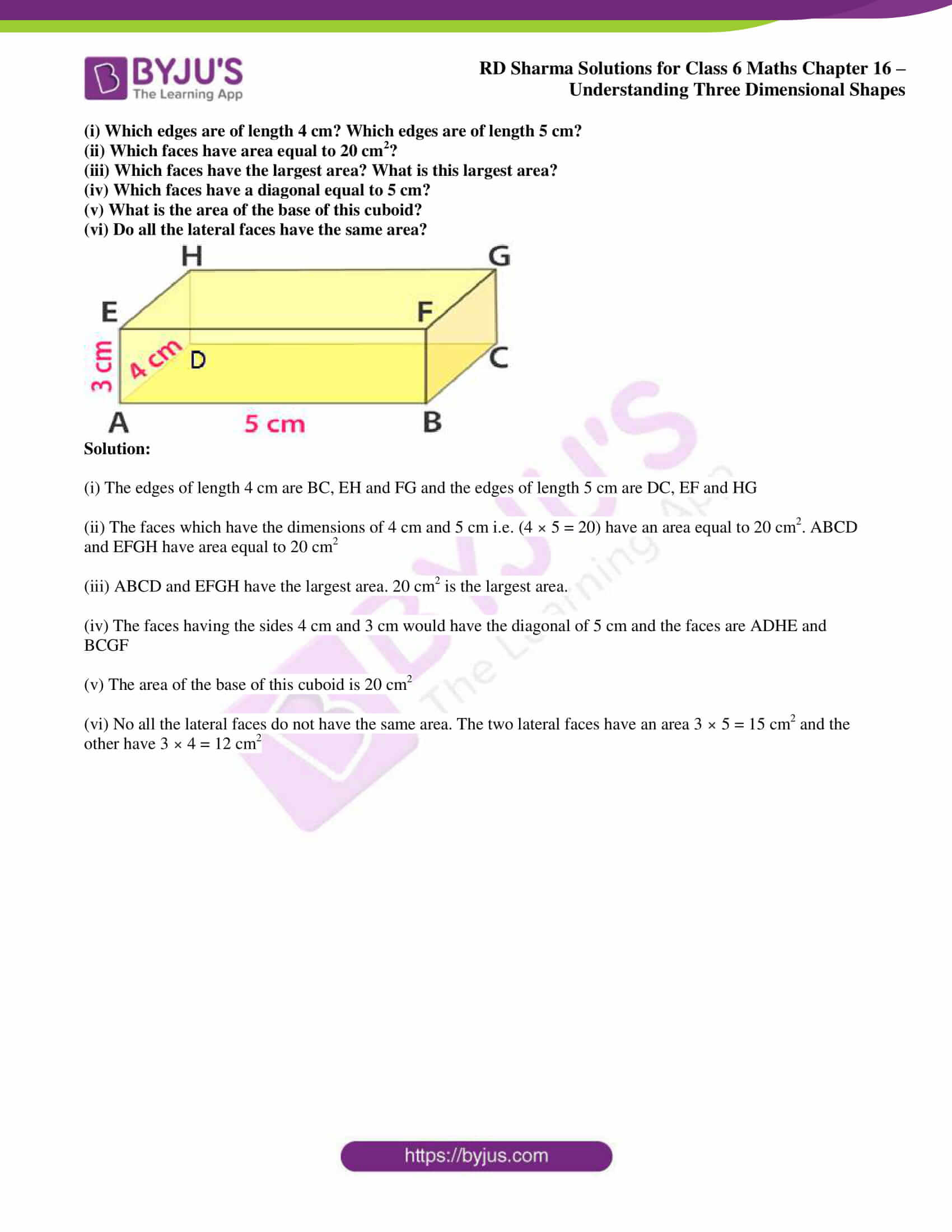### Exercise 16.1 page: 16.2

1. Name any four objects from your environment, which have the form of

(i) a cuboid (ii) a cube

Solution:

(i) The objects which have the form of a cuboid are a compass box, a lunch box, a duster and a book.

(ii) The objects which have the form of a cube are a chalk box, a disc, a tissue box and a cubical cabin.

2. Draw a diagram to represent a cuboid. Label its vertices as P, Q, R, S, T, U, V and W. Now write the names of its faces and its edges.

Solution:

The diagram of a cuboid is given below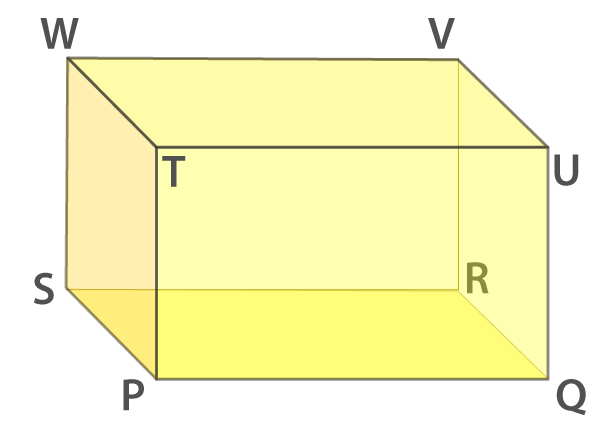The faces of a cuboid are

The bottom face – PQRS

The top face – TUVW

The back face – WSRV

The left face – TPSW

The right face – UVRQ

The front face – TPQU

The edges of a cuboid are

(PQ, QR, RS, SP), (TU, UV, VW, WT), (WS, SR, RV, VW) and (UV, VR, RQ, QU)

3. Draw a diagram to represent a cube. Label its vertices as A, B, C, D, E, F, G and H. Now write the names of its faces and edges.

Solution:

The diagram of a cube is as follows: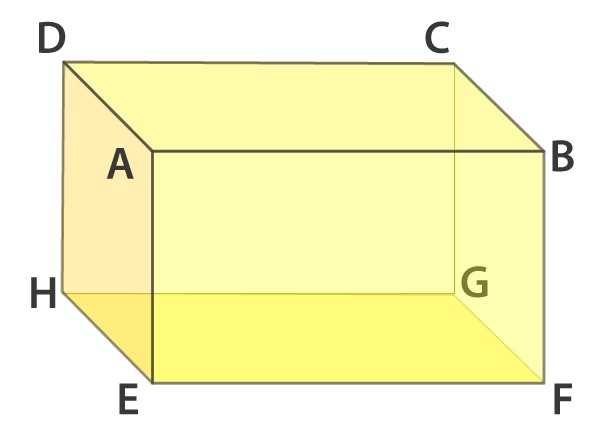We know that a cube has 6 faces and 12 edges.

The faces of a cube are

ABCD, EFGH, BCGF, ADHE, ABFE and CDHG.

The edges of a cube are

AB, BC, CD, DA, EF, FG, GH, HE, BF, CG, AE and HD

4. Fig. 16.2 represents a cuboid. The lengths of the edges AE, EF, and FG are indicated as l, b and h respectively. Indicated the lengths of all other edges.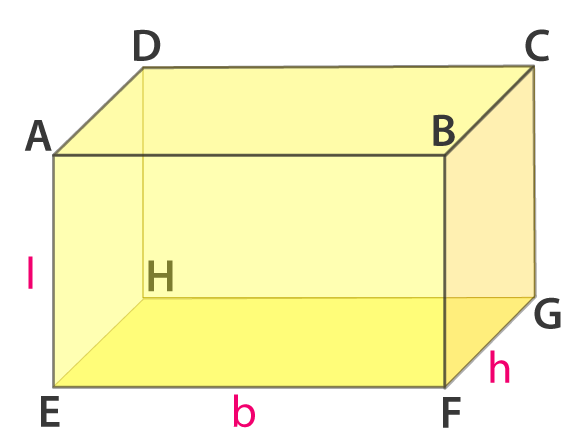Solution:

It is given that the lengths of the edges AE, EF and FG are indicated as l, b and h respectively. The lengths of all other edges are as follows

AE = DH = BF = CG = l

EF = HG = AB = DC = b

FG = EH = BC = AD = h

5. In Fig. 16.2, if the face EFGH is taken as the base, the name the lateral faces. Also, name the line segment representing the height of the cuboid.Solution:

The lateral faces for the base EFGH are

AEHD, AEFB, BFGC and DHGC

The line segments representing the height of the cuboid are

AE or DH or BF or CG

6. In Fig. 16.2, name the four diagonals of the cuboid.Solution:

CE, BH, AG and DF are the four diagonals of a cuboid.

7. In Fig. 16.2, name the

(i) face parallel to BFGC

(iii) three edges which meet in the vertex G.Solution:

(i) AEHD is the face parallel to BFGC

(ii) BCDA, DCGH, ABFE, and EFGH are the faces adjacent to BFGC

(iii) Three edges which meet in the vertex G are CG, GH and GF

8. Fill in the blanks to make the following statements true:

(i) A cuboid has …… vertices.

(ii) A cuboid has …… edges

(iii) A cuboid has ……. Faces.

(iv) The number of lateral faces of a cuboid is ……..

(v) A cuboid all of whose edges are equal is called a …….

(vi) Two adjacent faces of a cuboid meet in a line segment called its …….

(vii) Each edge of a cuboid can be obtained as a line segment in which two …… meet.

(viii) ……edges of a cube (or cuboid) meet at each of its vertices.

(ix) A ……. is a cuboid in which all the six faces are squares.

(x) The three concurrent edges of a cuboid meet at a point called the …… of the cuboid.

Solution:

(i) A cuboid has eight vertices

(ii) A cuboid has twelve edges

(iii) A cuboid has six faces

(iv) The number of lateral faces of a cuboid is four

(v) A cuboid all of whose edges are equal is called a cube

(vi) Two adjacent faces of a cuboid meet in a line segment called its edge

(vii) Each edge of a cuboid can be obtained as a line segment in which two adjacent faces meet

(viii) Three edges of a cube (or cuboid) meet at each of its vertices.

(ix) A cube is a cuboid in which all the six faces are squares

(x) The three concurrent edges of a cuboid meet at a point called the vertex of the cuboid.

9. In each of the following, state if the statement is true (T) or false (F):

(i) Number of faces in a cuboid and the number of faces in a cube are equal.

(ii) A cube has twelve vertices.

Solution:

(i) True

(ii) False

10. For the cuboid shown in Fig. 16.3,

(i) What is the base of this cuboid?

(ii) What are the lateral faces of this cuboid?

(iii) Name one pair of opposite faces. How many pairs of opposite faces are there? Name them.

(iv) Name all the faces of this cuboid which have X as a vertex. Also, name those which have VW as a side

(v) Name the edges of this cuboid which meet at the vertex P. Also, name those faces which meet at this vertex.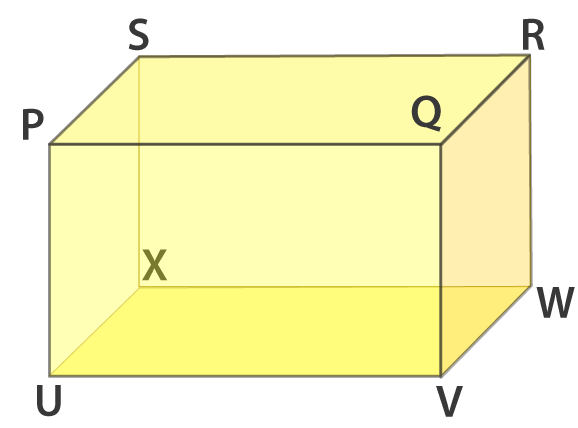Solution:

(i) The base of this cuboid is UVWX

(ii) The lateral faces of this cuboid are UXSP, QVWR, SXWR and UVQP

(iii) One pair of opposite faces among the lateral faces of the base are PQVU and SXWR or UXSP and QVWR. We know that a cuboid has two pairs of opposite faces among the lateral faces of the base.

(iv) The faces of this cuboid which have vertex as X are UVWX, UXSP and SXWR.

The faces having VW as a side are UVWX and QVWR

(v) The edges of this cuboid meeting at the vertex P are UP, PQ and PS.

The faces which meet at this vertex P are PQRS, UPSX and PQVU

11. The dimensions of a cuboid with vertices A, B, C, D, E, F, G and H are as shown in Fig. 16.4.

(i) Which edges are of length 4 cm? Which edges are of length 5 cm?

(ii) Which faces have area equal to 20 cm2?

(iii) Which faces have the largest area? What is this largest area?

(iv) Which faces have a diagonal equal to 5 cm?

(v) What is the area of the base of this cuboid?

(vi) Do all the lateral faces have the same area?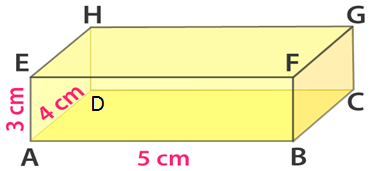Solution:

(i) The edges of length 4 cm are BC, EH and FG and the edges of length 5 cm are DC, EF and HG

(ii) The faces which have the dimensions of 4 cm and 5 cm i.e. (4 × 5 = 20) have an area equal to 20 cm2. ABCD and EFGH have area equal to 20 cm2

(iii) ABCD and EFGH have the largest area. 20 cm2 is the largest area.

(iv) The faces having the sides 4 cm and 3 cm would have the diagonal of 5 cm and the faces are ADHE and BCGF

(v) The area of the base of this cuboid is 20 cm2

(vi) No all the lateral faces do not have the same area. The two lateral faces have an area 3 × 5 = 15 cm2 and the other have 3 × 4 = 12 cm2